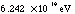# APPENDIX A

## The International System of Units

The International System of Units(SI), uses a single basic unit for each physical quantity, and multiples and fractions of this unit are formed by adding a prefix. For example, the SI unit for length is the meter (m). The millimeter (mm), centimeter (cm), and kilometer(km) are formed by adding the prefixes milli- (m), centi- (c), and kilo- (k) to meter. The same prefixes are used for all physical quantities. The prefixes and their symbols are listed in Table A.1.

The following rules should be observed when using SI symbols of units:

• Do not put a period after a symbol. Therefore, 7 km. is incorrect, but 7 km is correct.
• Do not put s at the end of any symbol. Therefore, 7kms is incorrect, but 7 km is correct.
• The symbol for a unit is a lowercase letter except when the unit is derived from a proper name(i.e. Newton, Pascal, Joule etc.). Thus, the symbol for the meter is m, whereas the symbol for the Newton is N. (An exception is the symbol for L for liter.)
• A symbol with the prefix is treated as a new unit which can be raised to a power without using brackets. Thus, the notation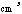means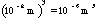, and not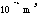.### Table A.1- SI Prefixes

 Prefix Symbol Value tera- T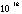giga- G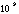mega- M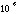kilo- k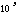hecto- h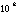deca- da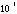deci- dcenti- c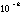milli- m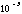micro-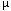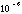nano- n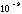pico- p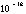femto- f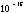atto- a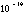### Table A.2- Common SI Units

#### Fundamental Units

 Quantity Name of Unit Symbol of Unit Length Meter m Mass Kilogram kg Time Second s Temperature Kelvin K Electric Current Ampere A

#### Derived Units

 Quantity Name of Unit Symbol of Unit Unit(with Fundamental Units) Frequency Hertz Hz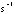Force Newton N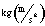Pressure Pascal Pa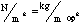Energy Joule J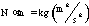Power Watt W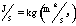Electric charge Coulomb C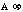Electric potential Volt V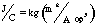Electric resistance Ohm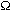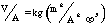Electric capacitance Farad F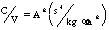Magnetic filed Tesla T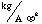Magnetic flux Weber W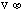### Table A.3- Conversion Factors

 Unit Conversion Units 1 degree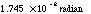1 revolution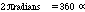1 meter 3.281ft=39.37in. 1 light year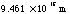1 kilogram 2.205lb=35.27oz 1 atomic mass unit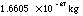1 slug 14.59kg 1 cubic meter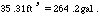1 joule